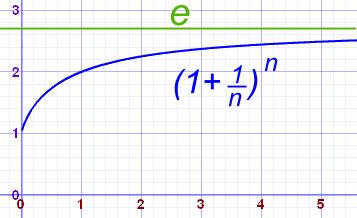# e (Euler's Number)The number e is one of the most important numbers in mathematics.

The first few digits are:

2.7182818284590452353602874713527 (and more ...)

It is often called Euler's number after Leonhard Euler (pronounced "Oiler").

e is an irrational number (it cannot be written as a simple fraction).

e is the base of the Natural Logarithms (invented by John Napier).

e is found in many interesting areas, so is worth learning about.

## Calculating

There are many ways of calculating the value of e, but none of them ever give a totally exact answer, because e is irrational and its digits go on forever without repeating.

But it is known to over 1 trillion digits of accuracy!

For example, the value of (1 + 1/n)n approaches e as n gets bigger and bigger:n (1 + 1/n)n 1 2.00000 2 2.25000 5 2.48832 10 2.59374 100 2.70481 1,000 2.71692 10,000 2.71815 100,000 2.71827

Try it! Put "(1 + 1/100000)^100000" into the calculator:

(1 + 1/100000)100000

What do you get?

## Another Calculation

The value of e is also equal to 10! + 11! + 12! + 13! + 14! + 15! + 16! + 17! + ... (etc)

(Note: "!" means factorial)

The first few terms add up to: 1 + 1 + 12 + 16 + 124 + 1120 = 2.71666...

In fact Euler himself used this method to calculate e to 18 decimal places.

You can try it yourself at the Sigma Calculator.

## Remembering

To remember the value of e (to 10 places) just remember this saying (count the letters!):

• To
• express
• e
• remember
• to
• memorize
• a
• sentence
• to
• memorize
• this

Or you can remember the curious pattern that after the "2.7" the number "1828" appears TWICE:

2.7 1828 1828

And following THAT are the digits of the angles 45°, 90°, 45° in a Right-Angled Isosceles Triangle (no real reason, just how it is):

2.7 1828 1828 45 90 45

(An instant way to seem really smart!)

## Growth

e is used in the "Natural" Exponential Function:Graph of f(x) = ex

It has this wonderful property: "its slope is its value"

At any point the slope of ex equals the value of ex :when x=0, the value ex = 1, and the slope = 1
when x=1, the value ex = e, and the slope = e
etc...

This is true anywhere for ex, and helps us a lot in Calculus when we need to find slopes etc.

So e is perfect for natural growth, see exponential growth to learn more.

## Area

The area up to any x-value is also equal to ex :## An Interesting Property

### Just for fun, try "Cut Up Then Multiply"

Let us say that we cut a number into equal parts and then multiply those parts together.

### Example: Cut 10 into 2 pieces and multiply them:

Each "piece" is 10/2 = 5 in size

5×5 = 25

Now, ... how could we get the answer to be as big as possible, what size should each piece be?

The answer: make the parts as close as possible to "e" in size.

### Example: 10

10 cut into 2 equal parts is 5:5×5 = 52 = 25
10 cut into 3 equal parts is 313:(313)×(313)×(313) = (313)3 = 37.0...
10 cut into 4 equal parts is 2.5:2.5×2.5×2.5×2.5 = 2.54 = 39.0625
10 cut into 5 equal parts is 2:2×2×2×2×2 = 25 = 32

The winner is the number closest to "e", in this case 2.5.

Try it with another number yourself, say 100, ... what do you get?

## 100 Decimal Digits

Here is e to 100 decimal digits:

2.71828182845904523536028747135266249775724709369995957
49669676277240766303535475945713821785251664274...

## Advanced: Use of e in Compound Interest

Often the number e appears in unexpected places. Such as in finance.

Imagine a wonderful bank that pays 100% interest.

In one year you could turn \$1000 into \$2000.

Now imagine the bank pays twice a year, that is 50% and 50%

Half-way through the year you have \$1500,
you reinvest for the rest of the year and your \$1500 grows to \$2250

You got more money, because you reinvested half way through.

That is called compound interest.

Could we get even more if we broke the year up into months?

We can use this formula:

(1+r/n)n

r = annual interest rate (as a decimal, so 1 not 100%)
n = number of periods within the year

Our half yearly example is:

(1+1/2)2 = 2.25

Let's try it monthly:

(1+1/12)12 = 2.613...

Let's try it 10,000 times a year:

(1+1/10,000)10,000 = 2.718...

Yes, it is heading towards e (and is how Jacob Bernoulli first discovered it).

### Why does that happen?

The answer lies in the similarity between:

 Compounding Formula: (1 + r/n)n and e (as n approaches infinity): (1 + 1/n)n

The Compounding Formula is very like the formula for e (as n approaches infinity), just with an extra r (the interest rate).

When we chose an interest rate of 100% (= 1 as a decimal), the formulas became the same.

## Euler's Formula for Complex Numbers

e also appears in this most amazing equation:

eiπ + 1 = 0

## Transcendental

e is also a transcendental number.

## e-DayCelebrate this amazing number on

• 27th January: 27/1 at 8:28 if you like writing your days first, or
• February 7th: 2/7 at 18:28 if you like writing your months first, or
• On both days!

2011, 2012, 2013